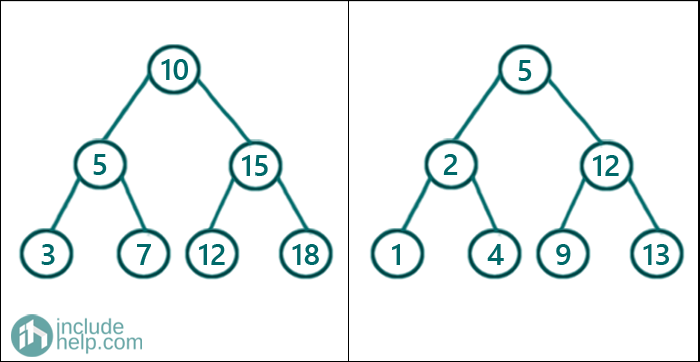# Merge Two Binary Search Trees Set 1

In this article, we are going to see how to merge two binary search trees?
Submitted by Radib Kar, on November 06, 2020

In this article, we are going to see how we can merge two binary search trees into one. Merging means collecting all the elements of two BSTs in a sorted fashion. So the output won't be a BST, rather sorted array based on the collection of elements from the two BSTs. For example,If we merge the above two BSTs then we will have the sorted collection as [1 2 3 4 5 5 7 9 10 12 12 13 15 18]

This is a very naïve solution where we will use multiset data structure. We will do an inorder traversal for both the trees one by one and the multiset will take care of ordering the elements in sorted order. Though the time complexity is O(n), there is an additional space requirement of O(2n).

Below is the C++ implementation:

```#include <bits/stdc++.h>
using namespace std;

// tree node is defined
class TreeNode {
public:
int val;
TreeNode* left;
TreeNode* right;
TreeNode(int data)
{
val = data;
left = NULL;
right = NULL;
}
};

void print(vector<int>& arr)
{
for (auto it : arr) {
cout << it << " ";
}
cout << endl;
}

void inorder(TreeNode* root, multiset<int>& result)
{
if (!root)
return;
inorder(root->left, result);
result.insert(root->val);
inorder(root->right, result);
}

vector<int> getAllElements(TreeNode* root1, TreeNode* root2)
{
multiset<int> result;
inorder(root1, result);
inorder(root2, result);
return vector<int>(result.begin(), result.end());
}

int main()
{
//trees built like the example
TreeNode* root1 = new TreeNode(10);
root1->left = new TreeNode(5);
root1->right = new TreeNode(15);
root1->left->left = new TreeNode(3);
root1->left->right = new TreeNode(7);
root1->right->left = new TreeNode(12);
root1->right->right = new TreeNode(18);

TreeNode* root2 = new TreeNode(5);
root2->left = new TreeNode(2);
root2->right = new TreeNode(12);
root2->left->left = new TreeNode(1);
root2->left->right = new TreeNode(4);
root2->right->left = new TreeNode(9);
root2->right->right = new TreeNode(13);

vector<int> mergedArr = getAllElements(root1, root2);

cout << "After merging two binary search trees, inorder traversal of the merged tree is:\n";
print(mergedArr);

return 0;
}
```

Output:

```After merging two binary search trees, inorder traversal of the merged tree is:
1 2 3 4 5 5 7 9 10 12 12 13 15 18
```

Though this is a naïve implementation, we have implemented to show the usage of multiset data structure.

Languages: » C » C++ » C++ STL » Java » Data Structure » C#.Net » Android » Kotlin » SQL
Web Technologies: » PHP » Python » JavaScript » CSS » Ajax » Node.js » Web programming/HTML
Solved programs: » C » C++ » DS » Java » C#
Aptitude que. & ans.: » C » C++ » Java » DBMS
Interview que. & ans.: » C » Embedded C » Java » SEO » HR
CS Subjects: » CS Basics » O.S. » Networks » DBMS » Embedded Systems » Cloud Computing
» Machine learning » CS Organizations » Linux » DOS
More: » Articles » Puzzles » News/Updates